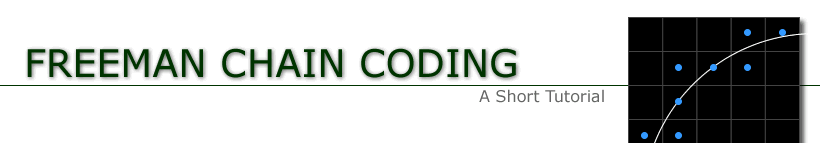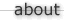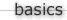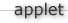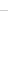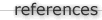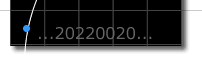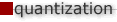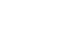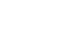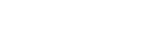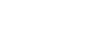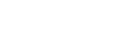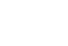Quantization Schemes

## Square Quantization

Each mesh node is taken to be the center of a square of side T as shown in the figure below. When some part of the line drawing or curve falls within the square, of a particular mesh node, that node is marked as a curve point. In the example of the curve given in the figure, this quantization scheme gives rise to the pattern with curve points shown in magenta.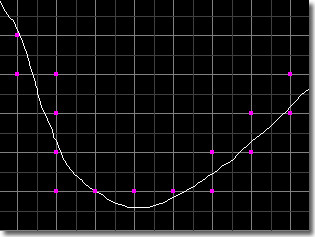## Circular Quantization

The same idea as above is applied in this quantization scheme except that each mesh node is considered to be the center of a cricle of radius T/2 as shown in the figure below. When some part of the line drawing falls within the circle, of which a mesh node is the center, that node is considered a curve point. This method gives rise to the sequence of curve points shown again in magenta in the figure below.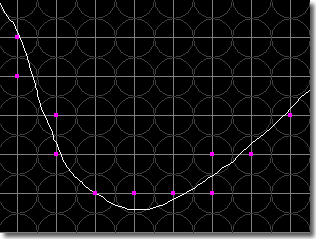## Grid-Intersect Quantization

In this scheme curve points are selected according to where the curve intersects the mesh lines between adjacent nodes. Each place the line drawing intersects the mesh, the mesh nodes associated with the mesh line are considered. The node which is closer to the intersection is selected as a curve point. When there is more than one intersection point around the mesh node, only one curve point is chosen. The sequence of magenta dots arises from this process as shown in the figure below.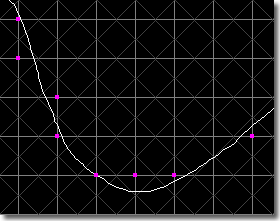The approximation of a line drawing using the sequence of curve points found using any of the three quantization schemes described above can be coded using at most 8 bits per line segment. This is because for any curve point the next curve point in the sequence can be in at most eight directions as shown in the figure below.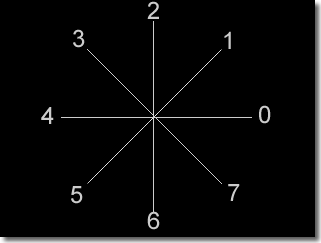Each line segment in the approximation is given a code according to the eight directions the segment can take, giving rise to a chain code representation for each line drawing. Each of the three quantization schemes desribed yield the chain codes shown in the next figure.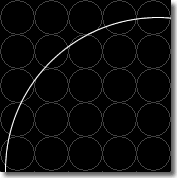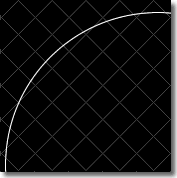Square Quantization Freeman Code 22020200 Circular Quantization Freeman Code 221010 Grid-Intersect Quantization Freeman Code 221100

The different quantization schemes often yield very different chain codes for the same line drawing. One of the factors in which the schemes differ is the number of diagonal line segments they contain. It can be readily seen that the square quantization does not give any diagonal segments (assuming that the line drawing never passes through the intersection of two mesh lines), whereas they do occur in circular and grid-intersect quantizations. According to Freeman, diagonal elements should occur half the time for random configurations for a good or close approximation.